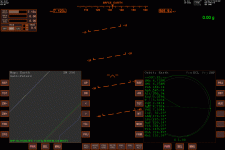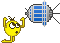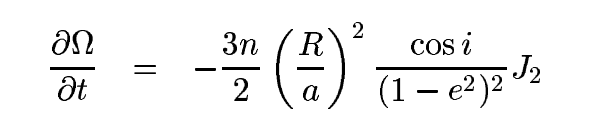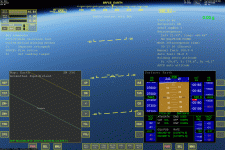# Non-spherical gravity for elliptic orbit

#### asbjos

##### tuanibrO
If one searches for the J2 contribution to change in longitude of the ascending node (LAN, $$\Omega$$) and argument of periapsis (APe$$\omega$$), the usual answer is some variation of
$\frac{\partial \Omega}{\partial t} = -3 \pi J_2 \left(\frac{R_E}{p}\right)^2 \cos i \cdot \frac{1}{T}$
$\frac{\partial \omega}{\partial t} = \frac{3}{2} \pi J_2 \left(\frac{R_E}{p}\right)^2 \left(5 \cos^2 i - 1\right) \cdot \frac{1}{T}$
with Earth radius $$R_E$$, equatorial inclination $$i$$, orbital period $$T$$ and semi-latus rectum $$p = a \sqrt{1 - e^2}$$ with semi-major axis $$a$$ and eccentricity $$e$$. So the change in LAN after e.g. 500 seconds is then:
$\Delta \Omega = -3 \pi J_2 \left(\frac{R_E}{p}\right)^2 \cos i \cdot \frac{500}{T}$.
This is even the equation given in the Orbiter Doc\Technotes\gravity.pdf.

This works well for circular orbits, or for integer multiples of orbits, but breaks down for elliptical orbits ($$0 < e < 1$$), or hyperbolic orbits ($$1 < e$$) for that matter. This is because the perturbation is proportional to the force of gravity, which itself is strongest at perigee and weakest at apogee.

I've looked online, and found these two publications: Kozai - Motion of a close Earth satellite and Sauer - Perturbations of a Hyperbolic Orbit. But I can't get either of them to work.Here, in my MFD map, the green is the unperturbed orbit, yellow is with time fraction, purple is with TrA fraction ($$\frac{\nu -\nu_0}{2 \pi}$$), red is Kozai and light gray is Sauer.
We can see that over three orbits, none of the implementations accurately predict the final position.

So the question is: is it at all possible to find the total change in the elements over the next $$t$$ seconds analytically? Or do I have to do some kind of iteration, and if so how?

The code of my implementation is
C++:
double J2 = oapiGetPlanetJCoeff(ref, 0); // 0 gives J2.
// "Default" fraction of orbital period:
if (perturbation == 1)
{
APe += 3.0 * PI2 / 4.0 * pow(refRad / el.a, 2.0) * (5.0 * cos(el.i) * cos(el.i) - 1.0) / pow(1.0 - el.e * el.e, 2.0) * J2 * t / prm.T;
LAN += -3.0 * PI2 / 2.0 * pow(refRad / el.a, 2.0) * cos(el.i) / pow(1.0 - el.e * el.e, 2.0) * J2 * t / prm.T;
}

// My adaptation with fraction of TrA
if (perturbation == 2)
{
double semiLatusRectum = el.a * (1.0 - el.e * el.e);
double TrA0 = prm.TrA;
APe += 3.0 * PI2 / 4.0 * refRad * refRad / semiLatusRectum / semiLatusRectum * (5.0 * cos(el.i) * cos(el.i) - 1.0) * J2 * (TrA - TrA0) / PI2;
LAN += -3.0 * PI2 / 2.0 * refRad * refRad / semiLatusRectum / semiLatusRectum * cos(el.i) * J2 * (TrA - TrA0) / PI2;
}

// Kozai:
if (perturbation == 3)
{
double sin2i = sin(el.i) * sin(el.i);
double e2 = el.e * el.e;
double semiLatusRectum = el.a / refRad * (1.0 - e2);
double meanMotion = PI2 / prm.T;
double semi2 = semiLatusRectum * semiLatusRectum;

double dOmegaS = -J2 / semi2 * cos(el.i) * (TrA0 - MnA + el.e * sin(TrA0) - 0.5 * sin(2.0 * (APe + TrA0)) - el.e / 2.0 * sin(TrA0 + 2.0 * APe) - el.e / 6.0 * sin(3.0 * TrA0 + 2.0 * APe)); // unsure if TrA0 and MnA0 or for time t.
double meanCos2TrA = (-el.e / (1.0 + sqrt(1.0 - e2))) * (-el.e / (1.0 + sqrt(1.0 - e2))) * (1.0 + 2.0 * sqrt(1.0 - e2)); // (11)
double meanDOmegaS = -1.0 / 6.0 * J2 / semi2 * cos(el.i) * meanCos2TrA * cos(2.0 * APe); // (12)
double OmegaDot = -J2 / semi2 * meanMotion * cos(el.i) * (1.0 + J2 / semi2 * (1.5 + e2 / 6.0 - 2.0 * sqrt(1.0 - e2) - sin2i * (5.0 / 3.0 - 5.0 / 24.0 * e2 - 3.0 * sqrt(1.0 - e2))));
double dOmega1 = meanDOmegaS - J2 / semi2 * e2 * cos(el.i) / (2.0 * (4.0 - 5.0 * sin2i)) * ((7.0 - 15.0 * sin2i) / 6.0 + 5.0 * sin2i / (2.0 * (4.0 - 5.0 * sin2i)) * (14.0 - 15.0 * sin2i) / 6.0) * sin(2.0 * APe);
LAN += OmegaDot * t + dOmegaS - meanDOmegaS + dOmega1;

double domegaS = J2 / semi2 * ((2.0 - 2.5 * sin2i) * (TrA - MnA + el.e * sin(TrA)) \
+ (1.0 - 1.5 * sin2i) * (1.0 / el.e * (1.0 - 0.25 * el.e) * sin(TrA) + 0.5 * sin(2.0 * TrA) + el.e / 12.0 * sin(3.0 * TrA)) \
- 1.0 / el.e * (0.25 * sin2i + (0.5 - 15.0 / 16.0 * sin2i) * e2) * sin(TrA + 2.0 * APe) + el.e / 16.0 * sin2i * sin(TrA - 2.0 * APe) \
- 0.5 * (1.0 - 2.5 * sin2i) * sin(2.0 * (TrA + APe)) + 1.0 / el.e * (7.0 / 12.0 * sin2i - 1.0 / 6.0 * (1.0 - 19.0 / 8.0 * sin2i) * e2) * sin(3.0 * TrA + 2.0 * APe) \
+ 3.0 / 8.0 * sin2i * sin(4.0 * TrA + 2.0 * APe) + el.e / 16.0 * sin2i * sin(5.0 * TrA + 2.0 * APe));
double meanDomegaS = J2 / semi2 * (sin2i * (0.125 + (1.0 - e2) / 6.0 / e2 * meanCos2TrA) + 1.0 / 6.0 * cos(el.i) * cos(el.i) * meanCos2TrA) * sin(2.0 * APe);
double omegaDot = J2 / semi2 * meanMotion * (2.0 - 2.5 * sin2i) * (1.0 + J2 / semi2 * (2.0 + e2 / 2.0 - 2.0 * sqrt(1.0 - e2) - sin2i * (43.0 / 24.0 - e2 / 48.0 - 3.0 * sqrt(1.0 - e2)))) - 5.0 / 12.0 * J2 * J2 / semi2 / semi2 * e2 * meanMotion * pow(cos(el.i), 4); // (28)
double domega1 = meanDomegaS - 3.0 / 8.0 * J2 / semi2 * sin2i * sin(2.0 * APe) - J2 / semi2 * (1.0 / (4.0 - 5.0 * sin2i) * ((14.0 - 15.0 * sin2i) / 24.0 * sin2i - e2 * (28.0 - 158.0 * sin2i + 135.0 * sin2i * sin2i) / 48.0) - e2 * sin2i * (13.0 - 15.0 * sin2i) / (4.0 - 5.0 * sin2i) / (4.0 - 5.0 * sin2i) * (14.0 - 15.0 * sin2i) / 24.0) * sin(2.0 * APe); // (30)
APe += omegaDot * t + domegaS - meanDomegaS + domega1; // (31)
}

// Sauer:
if (perturbation == 4)
{
double LANangleDiff = 6.0 * (TrA + el.e * sin(TrA)) - (3.0 * el.e * sin(2.0 * APe + TrA) + 3.0 * sin(2.0 * APe + 2.0 * TrA) + el.e * sin(2.0 * APe + 3.0 * TrA));
LANangleDiff -= 6.0 * (TrA0 + el.e * sin(TrA0)) - (3.0 * el.e * sin(2.0 * APe + TrA0) + 3.0 * sin(2.0 * APe + 2.0 * TrA0) + el.e * sin(2.0 * APe + 3.0 * TrA0));
double DeltaLAN = -J2 * cos(el.i) / 4.0 / pow(el.a / refRad * (1.0 - el.e * el.e), 2) * LANangleDiff; // we'll use this in the APe perturbation
LAN += DeltaLAN;

double APeAngleDiff = (1.0 - 1.5 * sin(el.i) * sin(el.i)) * (TrA + (4.0 + 3.0 * el.e * el.e) / (4.0 * el.e) * sin(TrA) + 0.5 * sin(2.0 * TrA) + el.e / 12.0 * sin(3.0 * TrA)) \
- sin(el.i) * sin(el.i) / (48.0 * el.e) * (3.0 * el.e * el.e * sin(2.0 * APe - TrA) + (12.0 - 21.0 * el.e * el.e) * sin(2.0 * APe + TrA) - 36.0 * el.e * sin(2.0 * APe + 2.0 * TrA) \
- (28.0 + 11.0 * el.e * el.e) * sin(2.0 * APe + 3.0 * TrA) - 18.0 * el.e * sin(2.0 * APe + 4.0 * TrA) - 3.0 * el.e * sin(2.0 * APe + 5.0 * TrA));
APeAngleDiff -= (1.0 - 1.5 * sin(el.i) * sin(el.i)) * (TrA0 + (4.0 + 3.0 * el.e * el.e) / (4.0 * el.e) * sin(TrA0) + 0.5 * sin(2.0 * TrA0) + el.e / 12.0 * sin(3.0 * TrA0)) \
- sin(el.i) * sin(el.i) / (48.0 * el.e) * (3.0 * el.e * el.e * sin(2.0 * APe - TrA0) + (12.0 - 21.0 * el.e * el.e) * sin(2.0 * APe + TrA0) - 36.0 * el.e * sin(2.0 * APe + 2.0 * TrA0) \
- (28.0 + 11.0 * el.e * el.e) * sin(2.0 * APe + 3.0 * TrA0) - 18.0 * el.e * sin(2.0 * APe + 4.0 * TrA0) - 3.0 * el.e * sin(2.0 * APe + 5.0 * TrA0));
APe += J2 * 3.0 / 2.0 / pow(el.a / refRad * (1.0 - el.e * el.e), 2) * APeAngleDiff - cos(el.i) * DeltaLAN;
}

I know this is a highly technical question, so I will allow myself to tag @MontBlanc2012 directly, as I assume that is one of the few who can give an answer. But I'm of course welcoming any answer from anybody else too.

•N_Molson

#### jarmonik

##### Well-known member
Orbiter Contributor
Beta Tester
Thanks, that looks very interesting. I have to study that more closely if I find some time to get back to MFD development someday.

I made some experiments regarding orbit stability like 15 years ago. If you compute Orbital Elements from a state vectors like they are computed in Orbiter.pdf this creates a case where a lot of fluctuation exists in SMa, PeA, PeT and so on.

But if you re-scale the "mu" to correspond actual simulation state the results become more stable. I don't kown if it's more or less correct way to do it. But I come-up with this equation for gravity compensated "mu":

$u_c = u - \frac{3uJ_2R_e^2(sin^2(Lat) - 1)}{4r^2}$

"R_e" is the equatorial radius of the Earth
"Lat" is current latitude

Last edited:

#### jarmonik

##### Well-known member
Orbiter Contributor
Beta Tester
Haven't you already used some kind of Runge-Kutta algorithm in the Travelmap MFD ? If analytic solutions are not accurate enough then Runge-Kutta is a good option.

This is a code I have use for nodel regression:
C:
    double J2  = oapiGetPlanetJCoeff(Ref, 0);
double Re  = oapiGetSize(Ref);
double inc = angle(_Rot, orbit->norv);

double xxx = Re*Re / (par*par);  // "par" is orbital parameter
double cos_inc = cos(inc);

double reg = -3.0 * J2 * mnm * xxx * cos_inc * time / 2.0;  // "mnm" is a mean motion
double aps =  3.0 * J2 * mnm * xxx * (5.0*cos_inc*cos_inc - 1) * time / 4.0;

I fail to see where is this coming from :
Code:
pow(1.0 - el.e * el.e, 2.0)
have you intended: pow(1.0 - el.e * el.e, 0.5)

Last edited:

#### asbjos

##### tuanibrO
Thanks for the replies!
I made some experiments regarding orbit stability like 15 years ago. If you compute Orbital Elements from a state vectors like they are computed in Orbiter.pdf this creates a case where a lot of fluctuation exists in SMa, PeA, PeT and so on.
Yes, I calculate the Kepler elements from state vectors, and the resulting elements are 1-to-1 with the ones shown in Orbit MFD. There's some fluctuation, yes, but I assume that is due to non-spherical gravity, n-body problem and air drag. Most importantly: if I disable non-spherical gravity and radiation pressure, the prediction is precisely correct.

But if you re-scale the "mu" to correspond actual simulation state the results become more stable. I don't kown if it's more or less correct way to do it. But I come-up with this equation for gravity compensated "mu":

$u_c = u - \frac{3uJ_2R_e^2(sin^2(Lat) - 1)}{4r^2}$
What do you mean by mu? Do you mean the standard gravitational parameter $$\mu = GM$$ or something else? And what is the $$u_c$$/$$u$$ here, is it the gravitational potential?

Haven't you already used some kind of Runge-Kutta algorithm in the Travelmap MFD ?
No, Travelmap MFD only displays recorded position vectors. There are no predictions in it, so it's a retrospective analogue to your map mode in IMFD, if you will.

I fail to see where is this coming from :
Code:
pow(1.0 - el.e * el.e, 2.0)
have you intended: pow(1.0 - el.e * el.e, 0.5)
It should be correct as it stands, as per the previous mentioned gravity technotes (page 3, eq. 7) and other sources:It's the same in the TrA analogue (perturbation == 2 case), but there I've bundled it into the semi-latus rectum $$p$$. Sorry for the inconsistencies. My brain is slowly melting over this problem, after countless papers of enormous trigonometric equations.As for numerical solutions to the problem, the physicist in me prefers exact analytical solutions (as can be done for the unperturbed case). But it sure does seem like a numerical solution is the only way.

Last edited:
•N_Molson

#### jarmonik

##### Well-known member
Orbiter Contributor
Beta Tester
What do you mean by mu? Do you mean the standard gravitational parameter $$\mu = GM$$ or something else? And what is the $$u_c$$/$$u$$ here, is it the gravitational potential?
Yes, by "mu" I mean $$\mu = GM$$ and in the equation above the $$\mu = u$$. $$u_c$$ is a non-spherical gravity scaled version of $$\mu$$
So, in other words if you compute gravitational acceleration effecting a vessel at a time "t" by using $$a = \mu/r^2$$ this doesn't include non-spherical gravity and the "a" is not exactly correct unless you are above the equator. But if the acceleration is computed from $$a = u_c/r^2$$ then the result should be the actual downwards gravitational pull at time "t". From my part this was just an educated guess that seems to work better. But I don't know if that is a right thing to do.

•asbjos

#### jarmonik

##### Well-known member
Orbiter Contributor
Beta Tester
Something in that equation I gave looks very wrong might be better to re-create it.

#### jarmonik

##### Well-known member
Orbiter Contributor
Beta Tester
From the Orbiter/Technotes/gravity.pdf

Downwards acceleration along the radius vector should be:
$a(r) = \frac{\mu}{r^2} - \frac{3\mu R^2J_2(3sin^2(\theta)-1)}{2r^4}$

Solving the $$\mu_c$$ that matches the acceleration
$\frac{\mu_c}{r^2} = \frac{\mu}{r^2} - \frac{3\mu R^2J_2(3sin^2(\theta)-1)}{2r^4}$
$\mu_c = \mu - \frac{3\mu R^2J_2(3sin^2(\theta)-1)}{2r^2}$

When computing osculating elements from a state vectors then which one actually should be used $$\mu$$ or $$\mu_c$$ ?

•N_Molson

#### jarmonik

##### Well-known member
Orbiter Contributor
Beta Tester
Here are some test results using "DG Mk4 in Orbit" scenario. Blue plot is using standard $$\mu$$ and the Orange is using compensated $$\mu_c$$ from the equation from the previous post. Interesting that "Orbit period" prediction is almost an exact opposite.
ApA = Apoapis altitude
PeA = Periapis altitude
LPe = Longitude of periapis
PeT = Error in "Time to periapis" prediction

"LAN" and "Inc" are identical in both cases.
Samples are taken 1 minute interval from periapis to periapis.

Of course, there is still a small gravity component in forward direction of flight that's not taken in account in anyway. Adding J3 terms has no effect.

Last edited:

#### jarmonik

##### Well-known member
Orbiter Contributor
Beta Tester
Out of curiosity I made an other test on equatorial orbit. Results are almost perfect.

For some reason following equation seems to work much better when computing mean-motion
$\mu_\eta(r,\theta) = \mu - \frac{3\mu R^2J_2(3sin^2(\theta)-1)}{4r^2}$
$\eta = \frac{|rv^2-2\mu_\eta|^{1.5}}{\mu_\eta r^{1.5}}$

#### Attachments

•asbjos

#### asbjos

##### tuanibrO
Thank you for all your replies, @jarmonik !
After much experimenting and back-and-forth, I implemented the RK4 propagation algorithm in the Map MFD.Yellow is RK4 spherical Earth (which fits perfectly with analytical spherical Earth), green is analytical non-spherical Earth J2 (using period fraction, which for this near-circular orbit is quite accurate), and blue is RK4 non-spherical Earth J2.
We can see that the green and blue (analytical non-spherical and RK4 non-spherical) are very similar, and both exaggerate the perturbation by roughly a factor 2.
I don't know why the J2 terms overshoots so much. I tried adding higher order J3 and J4, but that did not help. Also tried adding third body gravity from Moon/Sun, but that also didn't change much. I also tried your "adapted" $$\mu$$, but it didn't do much either.

So I'm a bit lost as to what is happening.

I also checked for a Molniya orbit (period 12 hours, inclination 63.4 degrees selected to eliminate drift from non-spherical perturbations).
The RK4 non-spherical is very stable, except for at perigee, where it shifts quickly. This compared to the RK4 spherical and analytical non-spherical solutions which shift over a longer time. Of course, the solution should not shift at all, although I prefer the generally more stable RK4 non-spherical.

In any case, the RK4 has a great benefit over the old analytical ground track prediction, in that the analytical broke down for highly spherical orbits (e < 0.01) or highly elliptical orbits (0.98 < e < 1.0), while the RK4 manages all eccentricities equally well.
Also, although I haven't checked, I imagine the RK4 solution is slightly less demanding on the processor, as it has almost no trigonometric functions, which the analytical has lots of.

••N_Molson and jarmonik

#### jarmonik

##### Well-known member
Orbiter Contributor
Beta Tester
Are you saying that RK4 non-speherical also fails to predict the position ? How much is the error ? If that's the case then I could try to tweak the LTMFD to see if it also drifts.

#### asbjos

##### tuanibrO
Are you saying that RK4 non-speherical also fails to predict the position ? How much is the error ? If that's the case then I could try to tweak the LTMFD to see if it also drifts.
I'm sorry for replying so late. I found a significant culprit: I had radiation pressure activated. With it activated, the error was 29 km after 4:45 hours in a 160x270 km orbit. With radiation pressure deactivated, the error is now 19 km.

And then I now reduced the time acceleration to max 100x (was previously at 1000x), the error is less than 200 m over 4:45 hours. So I guess my timesteps were so large that Orbiter automatically disabled non-spherical perturbation in its propagation method.

So false alarm, sorry for the inconvenience. And thanks for all the help.

Also the Molniya ground track is exceptionally accurate and stable if the time acc is max 100x during perigee.••jarmonik, kuddel and N_Molson